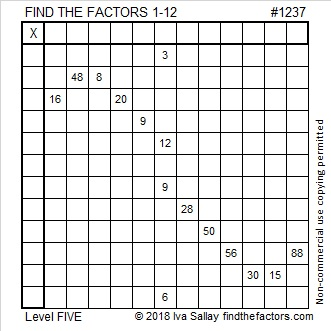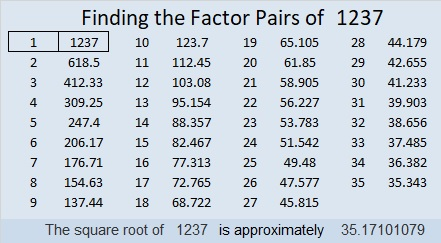# 1237 and Level 5

Level 5 puzzles always have at least one set of clues with more than one possible common factor. Still only one of those factors will actually work with all the rest of the clues. Can you use logic to find the factors needed to solve this puzzle?Print the puzzles or type the solution in this excel file: 12 factors 1232-1241

Let’s look at some facts about the number 1237:

• 1237 is a prime number.
• Prime factorization: 1237 is prime.
• The exponent of prime number 1237 is 1. Adding 1 to that exponent we get (1 + 1) = 2. Therefore 1237 has exactly 2 factors.
• Factors of 1237: 1, 1237
• Factor pairs: 1237 = 1 × 1237
• 1237 has no square factors that allow its square root to be simplified. √1237 ≈ 35.17101

How do we know that 1237 is a prime number? If 1237 were not a prime number, then it would be divisible by at least one prime number less than or equal to √1237 ≈ 35.1. Since 1237 cannot be divided evenly by 2, 3, 5, 7, 11, 13, 17, 19, 23, 29 or 31, we know that 1237 is a prime number.1237 is the sum of two squares:
34² + 9² = 1237

1237 is the hypotenuse of a Pythagorean triple:
612-1075-1237 calculated from 2(34)(9), 34² – 9², 34² + 9²

Here’s another way we know that 1237 is a prime number: Since its last two digits divided by 4 leave a remainder of 1, and 34² + 9² = 1237 with 34 and 9 having no common prime factors, 1237 will be prime unless it is divisible by a prime number Pythagorean triple hypotenuse less than or equal to √1237 ≈ 35.1. Since 1237 is not divisible by 5, 13, 17, or 29, we know that 1237 is a prime number.

This site uses Akismet to reduce spam. Learn how your comment data is processed.